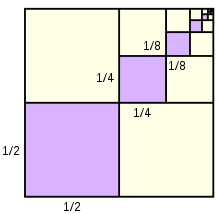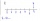# Calculate

Calculate the sum of all three-digit natural numbers divisible by five.

s =  98550

### Step-by-step explanation:Did you find an error or inaccuracy? Feel free to write us. Thank you!## Related math problems and questions:

• Find the sumFind the sum of all natural numbers from 1 and 100, which are divisible by 2 or 5
• Divisible by nineHow many three-digit natural numbers in total are divisible without a remainder by the number 9?
• Five-digit numberAnna thinks of a five-digit number that is not divisible by three or four. If he increments each digit by one, it gets a five-digit number that is divisible by three. If he reduces each digit by one, he gets a five-digit number divisible by four. If it sw
• Three-digit integersHow many three-digit natural numbers exist that do not contain zero and are divisible by five?
• 7 digit numberIf 3c54d10 is divisible by 330, what is the sum of c and d?
• Digit sumDetermine for how many integers greater than 900 and less than 1,001 has digit sum digit of the digit sum number 1.
• What are 3What are the two digits which when inserted in the blank spaces will make 234 _ _ divisible by 8?
• Sum of odd numbersFind the sum of all odd integers from 13 to 781.
• SymmetryEva loves symmetry in shapes and numbers. Yesterday she invented a completely new kind of symmetry - divisible symmetry. She wrote all five-digit numbers with different digits with the following property: the first digit is divisible by 1, the second by 2
• Twenty-fiveHow many are three-digit natural numbers divisible by 25?
• Sum of the seventeen numbersThe sum of the 17 different natural numbers is 154. Determine the sum of the two largest ones.
• Digit sumFind the smallest natural number n, the digit sum of which is equal to 37.
• The sumThe sum of the first 10 members of the arithmetic sequence is 120. What will be the sum if the difference is reduced by 2?
• Sum 1-6Find the sum of the geometric progression 3, 15, 75,… to six terms.
• Four-digit numbersFind four-digit numbers where all the digits are different. For numbers, the sum of the third and fourth digits is twice the sum of the first two digits, and the sum of the first and fourth digits is equal to the sum of the second and third digits. The di
• NumbersDetermine the number of all positive integers less than 4183444 if each is divisible by 29, 7, 17. What is its sum?
• Theorem proveWe want to prove the sentence: If the natural number n is divisible by six, then n is divisible by three. From what assumption we started?